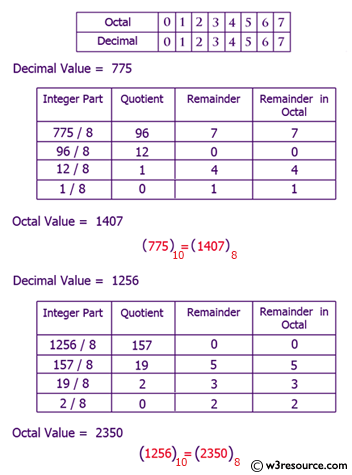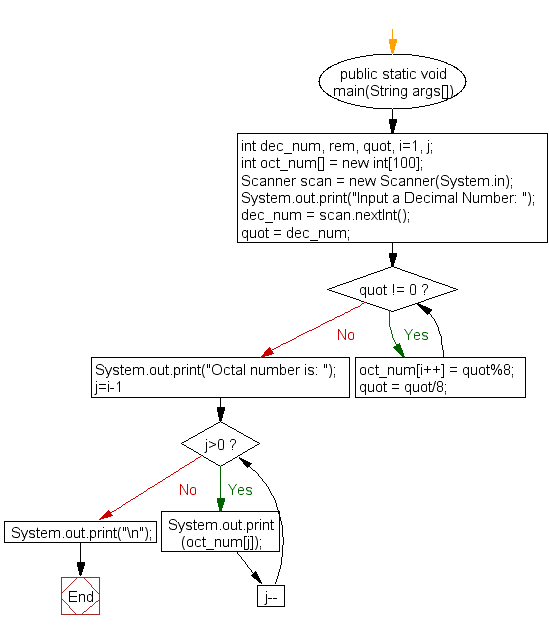﻿ Java exercises: Convert a decimal number to octal number - w3resource# Java Exercises: Convert a decimal number to octal number

## Java Basic: Exercise-21 with Solution

Write a Java program to convert a decimal number to octal number.

Decimal number: The decimal numeral system is the standard system for denoting integer and non-integer numbers. It is also called base-ten positional numeral system.

Octal number: The octal numeral system is the base-8 number system, and uses the digits 0 to 7.

Pictorial Presentation: Decimal to Octal numberSample Solution:

Java Code:

``````import java.util.Scanner;
public class Exercise21 {
public static void main(String args[])
{
int dec_num, rem, quot, i=1, j;
int oct_num[] = new int;
Scanner scan = new Scanner(System.in);

System.out.print("Input a Decimal Number: ");
dec_num = scan.nextInt();

quot = dec_num;

while(quot != 0)
{
oct_num[i++] = quot%8;
quot = quot/8;
}

System.out.print("Octal number is: ");
for(j=i-1; j>0; j--)
{
System.out.print(oct_num[j]);
}
System.out.print("\n");
}
}
```
```

Sample Output:

```Input a Decimal Number: 15
Octal number is: 17
```

Flowchart:Java Code Editor:

What is the difficulty level of this exercise?

Test your Programming skills with w3resource's quiz.

﻿

## Java: Tips of the Day

getEnumMap

Converts to enum to Map where key is the name and value is Enum itself.

```public static <E extends Enum<E>> Map<String, E> getEnumMap(final Class<E> enumClass) {
return Arrays.stream(enumClass.getEnumConstants())
.collect(Collectors.toMap(Enum::name, Function.identity()));
}
```

Ref: https://bit.ly/3xXcFZt